BLOG
Enjoy when you can, and endure when you must.MAR 12, 2014/Python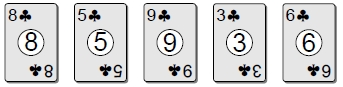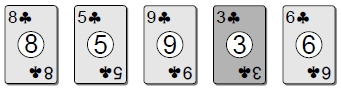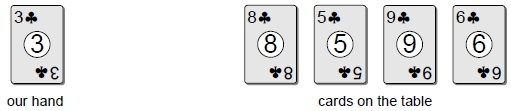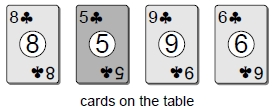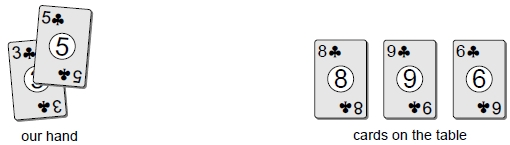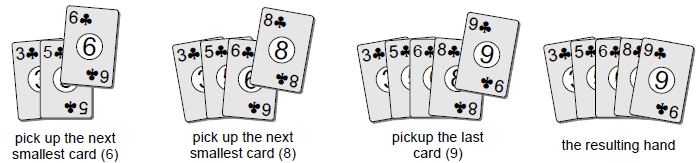```# Sorts a sequence in ascending order using the selection sort algorithm.
def selectionSort( theSeq ):
n = len( theSeq )
for i in range( n - 1 ):
# Assume the ith element is the smallest.
smallNdx = i
# Determine if any other element contains a smaller value.
for j in range( i + 1, n ):
if theSeq[j] < theSeq[smallNdx] :
smallNdx = j

# Swap the ith value and smallNdx value only if the smallest value is
# not already in its proper position. Some implementations omit testing
# the condition and always swap the two values.
if smallNdx != i :
theSeq[i], theSeq[smallNdx] = theSeq[smallNdx], theSeq[i]```

RECOMMENDS